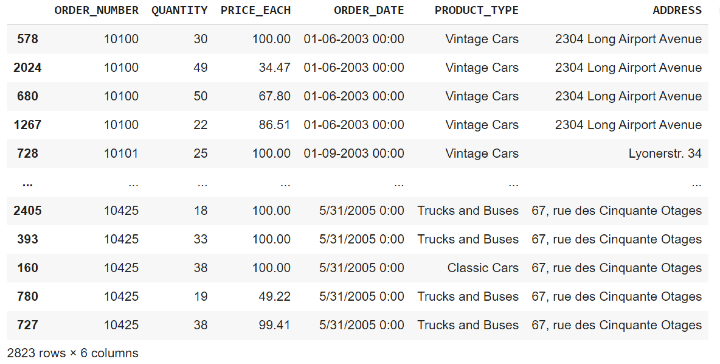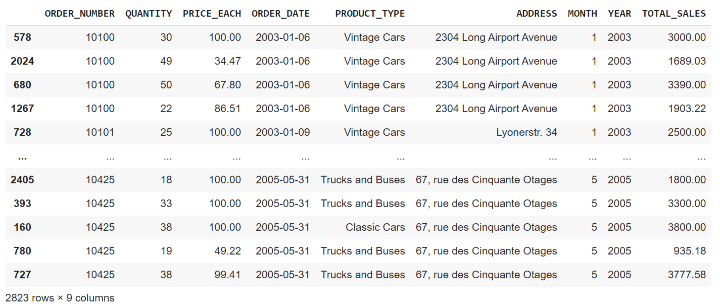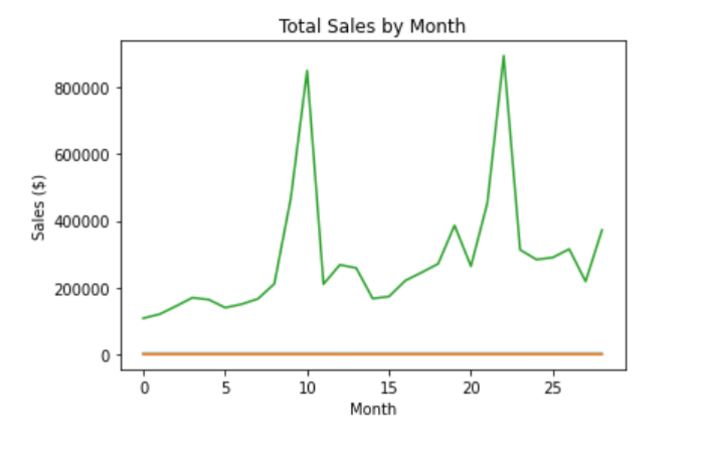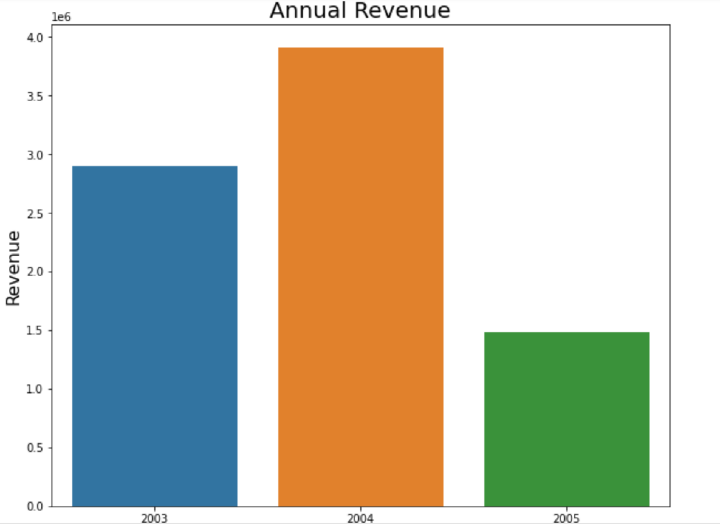# Active product sales analysis using matplotlib in Python

Matplotlib in Python has various functions like read_csv,sort_values,group_by, etc to perform sales data analysis. Every online business which is involved in product sales of any type uses product sales data analysis to increase their sales and know their customers better. Any company which is involved in any type of e-commerce business uses its sales and customer data to identify trends, patterns, and insights that can be used to improve sales and revenue. The sales data can be used to determine which product has the highest traction, which festive season has the highest demand, and many other trends which can help to increase sales.

Python is a popular programming language for data analysis and visualization. Python provides many libraries and tools that can be used to do product sales analysis effectively. In this article, we will use Matplotlib, which is a popular data visualization library in Python to do active product sales analysis.

We will be using sample sales data for active product sales analysis using numpy, pandas and matplotlib. The sample sales data can be found here.

## StepWise Sales Data Analysis

The sample sales data used in this example for analysis has the following columns −

 Order_Number Product_type Quantity Price_Each Order_Date Address
• Order Number − Unique number for each placed order.

• Product_Type − Category of the product

• Quantity − quantity of the product ordered

• Price Each − Price per unit

• Order Date − Date and time when the order was placed

We will have to import pandas and numpy which can be used to read and process the sample sales data. Here is the code to read the data −

The sample sales data can be found on the Kaggle platform here.

### Example

import pandas as pd
import numpy as np
import io

Sales_data.sort_values(by=['ORDER_NUMBER'])


### OutputOnce we have read the data, we have to do the processing of the data. The Order Date column needs to be converted to a DateTime object and we can extract month and year from the order date and add a new column for a month, year, and total sales. The code for data cleaning and processing is shown below −

### Example

Sales_data['ORDER_DATE'] = pd.to_datetime(Sales_data['ORDER_DATE'])
Sales_data['MONTH'] = Sales_data['ORDER_DATE'].dt.month
Sales_data['YEAR'] = Sales_data['ORDER_DATE'].dt.year
Sales_data['TOTAL_SALES'] = Sales_data['QUANTITY'] * Sales_data['PRICE_EACH']
Sales_data.sort_values(by=['ORDER_NUMBER'])


### OutputThe new column month, year, and toatal_sales will help us analyze the sales trend over time. Now we can use these columns to plot different plots using the matplotlib library to get some insights from the sample_sales_data.

### Data Visualization

Till now we have read and processed our data to use it to plot different plots using the matplotlib library in Python. Matplotlib provides line, bar, and scatter plots to visualize the data.

### Visualization of total sales over time

To visualize the total sales over time we can plot a line graph using matplotlib.To visualize that we have to −

• Group the data by year and month

• Create a line chart using matplotlib

• Set the title and axis labels

• Display the chart

### Example

import matplotlib.pyplot as plt

# Group the data by year and month
sales_by_month = Sales_data.groupby(['YEAR', 'MONTH']).sum()['TOTAL_SALES'].reset_index()

# Create a line chart
plt.plot(sales_by_month.index, sales_by_month.values)

# Set the title and axis labels
plt.title('Total Sales by Month')
plt.xlabel('Month')
plt.ylabel('Sales (\$)')

# Display the chart
plt.show()


### Output### Visualization of annual revenue over time

We can visualize the annual revenue for every year and can see which year has the highest revenue and which year has the lowest revenue till now. To do so we have to−

• Group the sales data by year

• Create a bar plot using Seaborn which uses matplotlib underneath

• Set the title and axis labels

• Display the chart

### Example

import numpy as np
import pandas as pd
import matplotlib.pyplot as plt
import seaborn as sns

# Annual Revenue
plt.figure(figsize=(10,6))
yearly_revenue = Sales_data.groupby(['YEAR'])['TOTAL_SALES'].sum().reset_index()
sns.barplot(x="YEAR", y="TOTAL_SALES", data=yearly_revenue)

plt.title('Annual Revenue', fontsize = 20)
plt.xlabel('Year', fontsize = 16)
plt.ylabel('Revenue', fontsize = 16)

plt.show()


### Output## Conclusion

We can analyze and visualize any type of product sales data using matplotlib in Python and get standard data insights that can be used by the company to increase sales. We analyzed the total sales over time and year-wise revenue in the above article using matplotlib, pandas, and numpy in Python.

Updated on: 17-Apr-2023

639 Views